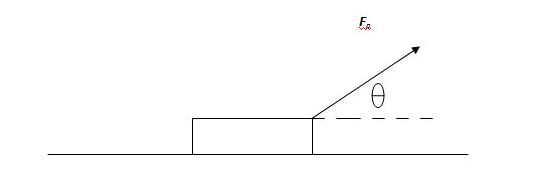PH 220 Midterm Exam 100% Correct Answers

( 1 ) Three forces pull on a block, the first is 65 N and in the positive x direction. The second is 65 N in the negative x direction and the third is 45 N and is 90 degrees from the first two forces in the positive y direction. What can you say about the resultant force?

( 2 ) A cannonball is fired horizontally with an initial velocity of 655 m/s from the end of a cliff 725 m high. How far in the x direction does the cannonball in the air before it hits the ground?

( 3 ) The international space station orbits the Earth at an altitude of about 250 km. What is the acceleration due to gravity at that height above the ground? The mass of the Earth is 5.98 x 1024 kg and the radius of the Earth is 6371 km.

( 4 ) A subatomic particle with mass m initially are rest suddenly decays. If one of the resultant particles with mass 0.40 m of mass leaves with a velocity of 5.5 x 105 m/s, what is the velocity of the other particle created?

( 5 ) A 7.93 kg box is pulled along a horizontal surface by a force FP of 84.0 N applied at a 47.0º angle. If the coefficient of kinetic friction is 0.35, what is the acceleration of the box?( 6 ) A car uniformly decelerates at a rate of -5.00 m/s2. How far does the car travel after 1.50 seconds if it initially was moving at a speed of 25.0 m/s?

( 7 ) A 25 kg is acted on by a 45 N horizontal force. If the box accelerates at a rate of .75 m/s2, what is the coefficient of kinetic friction between the box and the table?

( 8 ) A ball is at the top of a ramp that is 4.5 m above a table. If the balls rolls without friction, how fast is it going at the bottom of the ramp?